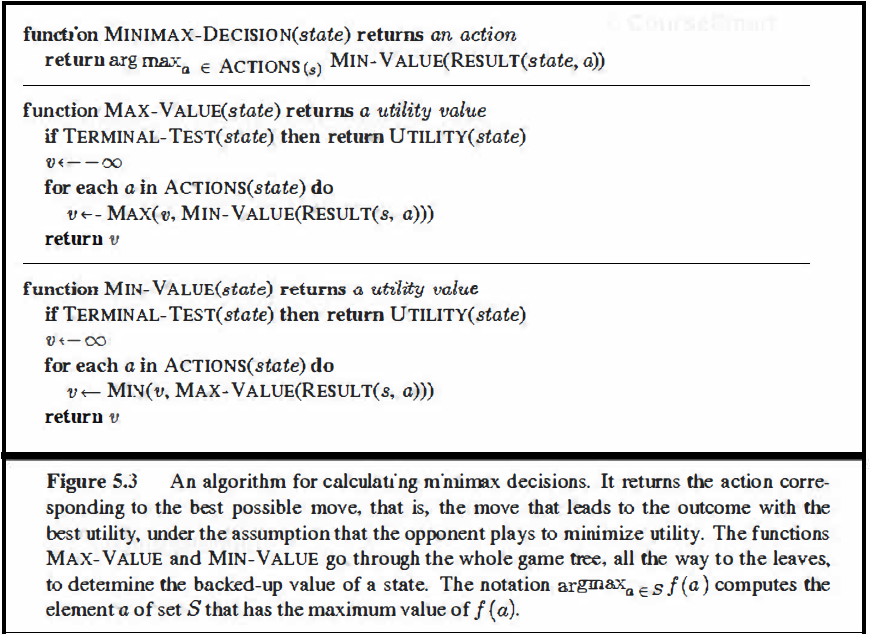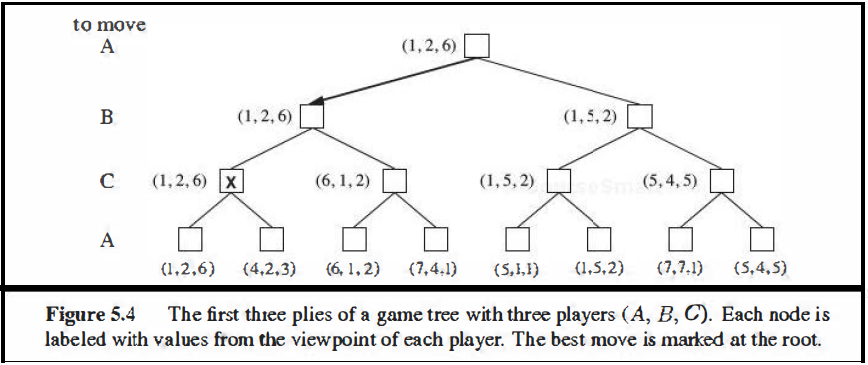The minimax algorithm: The minimax algorithm (Figure 5.3) computes the minimax decision from the current state. It uses a simple recursive computation of the minimax values of each successor state, directly implementing the defining equations. The recursion proceeds all the way down to the leaves of the tree, and then the minimax values are backed up through the tree as the recursion unwinds. For example, in Figure 5.2, the algorithm first recurses down to the three bottomleft nodes and uses the UTILITY function on them to discover that their values are 3, 12, and 8, respectively. Then it takes the minimum of these values, 3, and returns it as the backedup value of node B. A similar process gives the backed-up values of 2 for C and 2 for D. Finally, we take the maximum of 3, 2, and 2 to get the backed-up value of 3 for the root node.

The minimax algoritlun performs a complete depth-first exploration of the game tree. If the maximum depth of the tree is m and there are b legal moves at each point, then the time complexity of the minimax algoritlun is O(b m). The space complexity is O(lnn) for an algorithm that generates all actions at once, or 0( m) for an algorithm that generates actions one at a time. For real games, of course, the time cost is totally impractical, but this algorithm serves as the basis for the mathematical analysis of games and for more practical algorithms.

Optimal decisions in multiplayer games: Many popular games allow more than two players. Let us examine how to extend the minimax idea to multiplayer games. This is straightforward from the technical viewpoint, but raises some interesting new conceptual issues.

First, we need to replace the single value for each node with a vector of values. For example, in a three-player game with players A, B, and C, a vector (vA, VB, vc) is associated with each node. For terminal states, this vector gives the utility of the state from each player’s viewpoint. (In two-player, zero-sum games, the two-element vector can be reduced to a single value because the values are always opposite.) The simplest way to implement this is to have the UTILITY function return a vector of utilities.
Now we have to consider non terminal states. Consider the node marked X in the game tn::t: shown in Figure 5.4. In that state player C what to do. The two Choice lead to terminal states with utility vectors (vA = 1, VB= 2, vc = 6) and (vA = 4, VB= 2, vc = 3). Since 6 is bigger than 3, C should choose the first move. This means that if state X is reached, subsequent play will lead to a terminal state with utilities (vA = 1, VB= 2, vc = 6). Hence, the backed-up value of X is this vector. The backed-up value of a node n is always the utilityvector of the successor state with the highest value for the player choosing at n. Anyone who plays multiplayer games, such as Diplomacy, quickly becomes aware that much more is going on than in two-player games. Multiplayer games usually involve alliances, whether formal or informal, among the players. Alliances are made and broken as the game proceeds. How are we to understand such behavior? Are alliances a natural consequence of optimal strategies for each player in a multiplayer game? It turns out that they can be. For example, suppose A and B are in weak positions and C is in a stronger position. Then it is often optimal for both A and B to attack C rather than each other, lest C destroy each of them individually. In this way, collaboration emerges from purely selfish behavior. Of course, as soon as C weakens under the joint onslaught, the alliance loses its value, and either A or B could violate the agreement. In some cases, explicit alliances merely make concrete what would have happened anyway. In other cases, a social stigma attaches to breaking an alliance, so players must balance the immediate advantage of breaking an alliance against the long-term disadvantage of being perceived as untrustworthy. See Section 17.5 for more on these complications.
If the game is not zero-sum, then collaboration can also occur with just two players. Suppose, for example, that there is a terminal state with utilities (vA = 1000, VB= 1000) and that 1000 is the highest possible utility for each player. Then the optimal strategy is for both players to do everything possible to reach this state-that is, the players will automatically cooperate to achieve a mutually desirable goal.